• ## 循环矩阵傅里叶对角化

万次阅读 多人点赞 2016-03-15 14:10:30
“任意循环矩阵可以被傅里叶变换矩阵对角化”这个概念常常出现在论文中，本文其做简单解释。

All circulant matrices are made diagonal by the Discrete Fourier Transform (DFT), regardless of the generating vector x.
任意循环矩阵可以被傅里叶变换矩阵对角化。

文献中，一般用如下方式表达这一概念：
$X=C(x)=F \cdot diag(\hat{x}) \cdot F^H$
其中$X$是循环矩阵，$\hat{x}$是原向量$x$的傅里叶变换，$F$是傅里叶变换矩阵，上标H表示共轭转置：$X^H=(X^{*})^T$。
换句话说，$X$相似于对角阵，$X$的特征值是$\hat x$的元素。
另一方面，如果一个矩阵能够表示成两个傅里叶矩阵夹一个对角阵的乘积形式，则它是一个循环矩阵。其生成向量是对角元素的傅里叶逆变换：
$F \cdot diag(y) \cdot F^H = C(\mathcal{F}^{-1}(y))$
这个公式初看疑问很多，以下一一讨论。
$X$是什么？
$X$是由原向量$x$生成的循环矩阵。以矩阵尺寸$K=4$为例。
$X=C(x)=\begin{bmatrix} x_1 & x_2 & x_3 & x_4 \\ x_4 & x_1 & x_2 & x_3 \\ x_3 & x_4 & x_1 & x_2 \\ x_2 & x_3 & x_4 & x_1 \\ \end{bmatrix}$
$F$ 是什么？
$F$是离散傅里叶矩阵（DFT matrix），可以用一个复数$\omega = e^{-2\pi i/K}$表示，其中$K$为方阵$F$的尺寸。以$K=4$为例。
$F=\frac{1}{\sqrt{K}} \begin{bmatrix} 1 & 1 & 1 & 1 \\ 1 & \omega & \omega^2 & \omega^3 \\ 1 & \omega^2 & \omega ^4 & \omega^6 \\ 1 & \omega^3 & \omega^6 & \omega^9 \end{bmatrix}$
把$\omega$想象成一个角度为$2\pi/K$的向量，这个矩阵的每一行是这个向量在不断旋转。从上到下，旋转速度越来越快。
之所以称为DFT matrix，是因为一个信号的DFT变换可以用此矩阵的乘积获得：
$\hat{x}=DFT(x)=\sqrt{K}\cdot F \cdot x$
反傅里叶变换也可以通过类似手段得到：
$x=\frac{1}{\sqrt{K}}F^{-1}\hat{x}$
傅里叶矩阵有许多性质：

是对称矩阵，观察$\omega$的规律即可知；
满足$F^HF=FF^H=I$，也就是说它是个酉矩阵（unitary）。可以通过将$F^{H}$展开成$\omega^{H}$验证。

注意：$F$是常数，可以提前计算好，和要处理的$x$无关。
对角化怎么理解？
把原公式两边乘以逆矩阵：
$F^{-1} \cdot X \cdot (F^H)^{-1}=diag(\hat{x})$
利用前述酉矩阵性质：
$左边=F^{H}XF=diag(\hat{x})$
也就是说，矩阵$X$通过相似变换$F$变成对角阵$diag(\hat{x})$，即对循环矩阵$X$进行对角化。
另外，$F^HXF$是矩阵$X$的2D DFT变换。即傅里叶变换可以把循环矩阵对角化。
怎么证明？
可以用构造特征值和特征向量的方法证明（参看这篇论文1的3.1节），此处简单描述。
考察待证明等式的第k列：
$X\cdot f_k=\hat x_k\cdot f_k$
其中$f_k$表示DFT矩阵的第k列，$\hat x_k$表示傅里叶变换的第k个元素。等价于求证：$f_k$和$\hat x_k$是$X$的一对特征向量和特征值。
左边向量的第i个元素为：$left_i = \left[ x^i, f_k \right]$。$x_i$表示把生成向量$x$向右移动i位，$[]$表示内积。
内积只和两个向量的相对位移有关，所以可以把$f_k$向左移动i位：$left_i=\left[ x, f_k^{-i}\right]$。
DFT矩阵列的移位可以通过数乘$\omega$的幂实现：$f_k^i=f_0\cdot \omega^{ik}$。

举例：$K=3$，
$F=\frac{1}{\sqrt{K}} \begin{bmatrix} 1 & 1 & 1\\ 1 & \omega & \omega^2 \\ 1 & \omega^2 & \omega ^4 \end{bmatrix}$

利用$\omega^N=1$.
$f_1\cdot \omega =[1,\omega,\omega^2]\cdot \omega = [\omega, \omega^2,\omega^3] = [\omega, \omega^2, 1]=f_1^{-1}$

$f_1\cdot \omega^2 =[1,\omega,\omega^2]\cdot \omega^2 = [\omega^2, \omega^3,\omega^4] = [\omega^2, 1, \omega]=f_1^{-2}$

于是有：
$left_i= \left [x, f_k \cdot \omega^{ik}\right ]=\left [x, f_k\right ] \cdot \omega^{ik}$
右边的$\hat x = F\cdot x $，考虑到$F$的第k行和第k列相同，$\hat x_k=[f_k,x]$。另外$f_k$的第i个元素为$\omega^{ik}$：
$right_i = \hat x_k\cdot f_{ki}=[f_k^*,x]\cdot \omega^{ki}$
对任意k列的第i个元素有：$left_i=right_i$
更多性质
利用对角化，能推导出循环矩阵的许多性质。
转置
循环矩阵的转置也是一个循环矩阵（可以查看循环矩阵各元素排列证明），其特征值和原特征值共轭。
$X^T=F \cdot diag((\hat{x})^*) \cdot F^H$
可以通过如下方式证明：
$X^T=(F^H)^T\cdot diag(\hat x) F^T$
由于$F$是对称酉矩阵，且已知$X$是实矩阵：
$X^T=F^*\cdot diag(\hat x) F=\left( F^*\cdot diag(\hat x) F\right)^*=F\cdot diag((\hat x)^*) \cdot F^H$
如果原生成向量$x$是对称向量（例如[1,2,3,4,3,2]），则其傅里叶变换为实数，则：
$X^T=C\left( \mathcal F^{-1}(\hat x)^* \right)=C(x)$
卷积
循环矩阵乘向量等价于生成向量的逆序和该向量卷积，可进一步转化为福利也变化相乘。
注意卷积本身即包含逆序操作，另外利用了信号与系统中经典的“时域卷积，频域相乘”。
$\mathcal{F}(Xy) = \mathcal{F}(C(x)y)=\mathcal{F}(\bar x*y)=\mathcal{F}^*(x)\odot \mathcal{F}(y)$
其中$\bar x$表示把$x$的元素倒序排列。星号表示共轭。
相乘
设$C,B$为循环矩阵，其乘积的特征值等于特征值的乘积：
$AB = F\cdot diag(\hat a) \cdot F^H \cdot F \cdot diag(\hat b) \cdot F^H$
$= F\cdot diag(\hat a) \cdot diag(\hat b) \cdot F^H=F\cdot diag(\hat a \odot \hat b) \cdot F^H$
$=C\left( \mathcal F^{-1}(\hat a \odot \hat b)\right)$
乘积也是循环矩阵，其生成向量是原生成向量对位相乘的傅里叶逆变换。
相加
和的特征值等于特征值的和：
$A+B = F\cdot diag(\hat a) \cdot F^H + F \cdot diag(\hat b) \cdot F^H=F\cdot diag(\hat a +\hat b) \cdot F^H$
$=C\left( \mathcal F^{-1} (\hat a +\hat b)\right)=C\left( \mathcal F^{-1} (\hat a )+F^{-1} (\hat b )\right)=C\left( a+b\right)$
和也是循环矩阵，其生成向量是原生成向量的和。
求逆
循环矩阵的逆，等价于将其特征值求逆。
$X^{-1}=F\cdot diag(\hat{x})^{-1}F^H=C\left( \mathcal F^{-1}(diag(\hat{x})^{-1}) \right)$
对角阵求逆等价于对角元素求逆。以下证明：
$F\cdot diag(\hat{x})^{-1}\cdot F^H \cdot F\cdot diag(\hat{x}) \cdot F^H$
$=F\cdot diag(\hat{x})^{-1}\cdot diag(\hat{x}) \cdot F^H=F\cdot F^H=I$
逆也是循环矩阵
有什么用？
该性质可以将循环矩阵的许多运算转换成更简单的运算。例如：
$X^HX=F \cdot diag(\hat{x} \odot \hat{x}^*)\cdot F^H=C\left( \mathcal F^{-1}(\hat{x} \odot \hat{x}^*) \right)$
原始计算量：两个方阵相乘（$O(K^3)$）
转化后的计算量：反向傅里叶($K\log K$)+向量点乘（$K$）。
CV的许多算法中，都利用了这些性质提高运算速度，例如2015年TPAMI的这篇高速跟踪KCF方法2。
二维情况
以上探讨的都是原始信号为一维的情况。以下证明二维情况下的$F^HXF=diag(\hat x)$，推导方法和一维类似。
$x$是二维生成矩阵，尺寸$N\times N$。
$X$是一个$N^2\times N^2$的分块循环矩阵，其uv块记为$x^{uv}$，表示$x$下移u行，右移v列。
$F$是$N^2\times N^2$的二维DFT矩阵，其第uv块记为$f_{uv}=\left\{ \omega^{ui+vj} \right\} _{ij}$。

举例：N=3 $f_{01}=\begin{bmatrix} 1 & \omega & \omega^2 \\ 1 & \omega & \omega^2 \\ 1 & \omega & \omega^2\end{bmatrix}, f_{11}=\begin{bmatrix} 1 & \omega & \omega^2 \\ \omega & \omega^2 & \omega^3 \\ \omega^2 & \omega^3 & \omega^4\end{bmatrix}\\$

需要验证的共有$N\times N$个等式，其中第$uv$个为：
$[X, f_{uv}]=\hat x_{uv}\cdot f_{uv}$
其中$[X, f_{uv}]$表示把$x^{uv}$分别和$f_{uv}$做点乘，结果矩阵元素求和。
这个等式的第ij元素为：
$[x^{ij},f_{uv}]=\hat x_{uv} \cdot \omega^{ui+vj}$
再次利用两个性质：1) 点乘只和两个矩阵相对位移有关，2) $f_{uv}$的位移可以用乘$\omega$幂实现：
$left_{ij}=[x,f_{uv}^{-i-j}]=[x,f_{uv}]\cdot \omega^{ui+vj}=\hat x_{uv} \cdot \omega^{ui+vj}=right_{ij}$
代码
以下matlab代码验证上述性质。需要注意的是，matlab中的dftmatx函数给出的结果和本文定义略有不同，需做一简单转换。另外，matlab中的撇号表示共轭转置，transpose为转置函数，conj为共轭函数。
clear;clc;close all;

% 1. diagnolize
K = 5;      % dimension of problem

x_base = rand(1,K);     % generator vector
X = zeros(K,K);         % circulant matrix
for k=1:K
X(k,:) = circshift(x_base, [0 k-1]);
end

x_hat = fft(x_base);    % DFT

F = transpose(dftmtx(K))/sqrt(K);       % the " ' " in matlab is transpose + conjugation

X2 = F*diag(x_hat)*F';

display(X);
display(real(X2));

% 2. fast compute correlation
C = X'*X;
C2 = (x_hat.*conj(x_hat))*conj(F)/sqrt(K);

display(C);
display(C2);


Gray, Robert M. Toeplitz and circulant matrices: A review. now publishers inc, 2006. ↩︎

Henriques, João F., et al. “High-speed tracking with kernelized correlation filters.” Pattern Analysis and Machine Intelligence, IEEE Transactions on 37.3 (2015): 583-596. ↩︎


展开全文傅里叶变换
• 文章类容根据《Matrix Analysis and Applied Linear Alebra》第5.8章节内容翻译。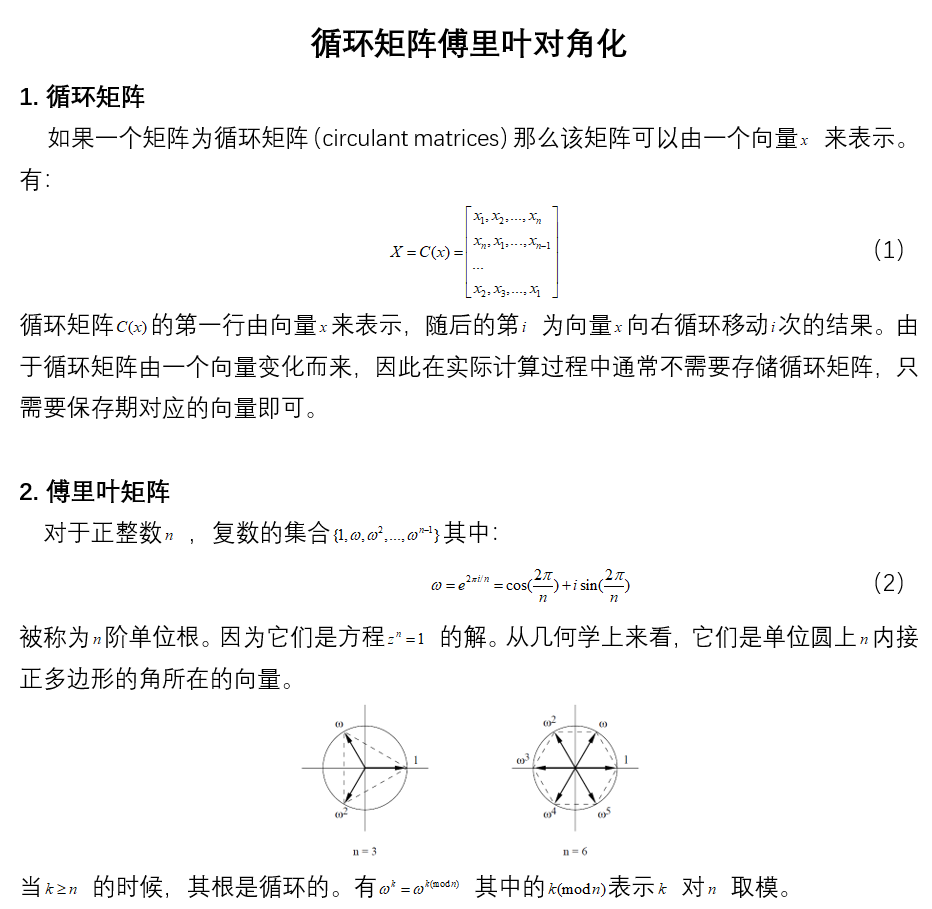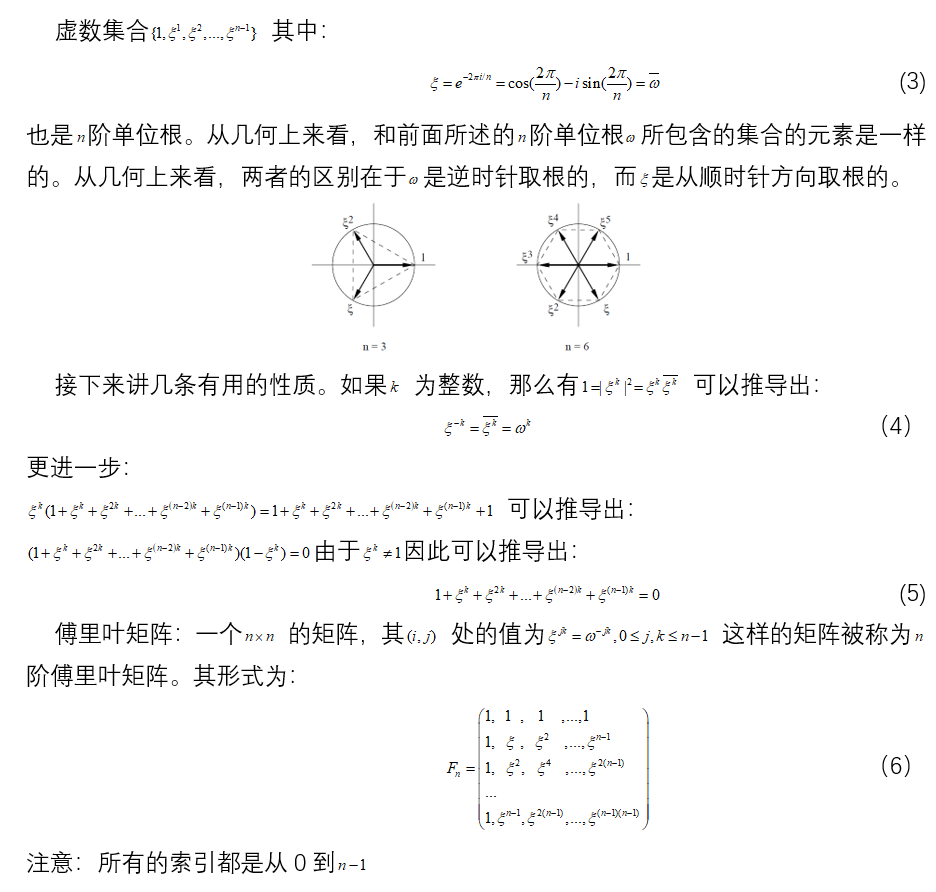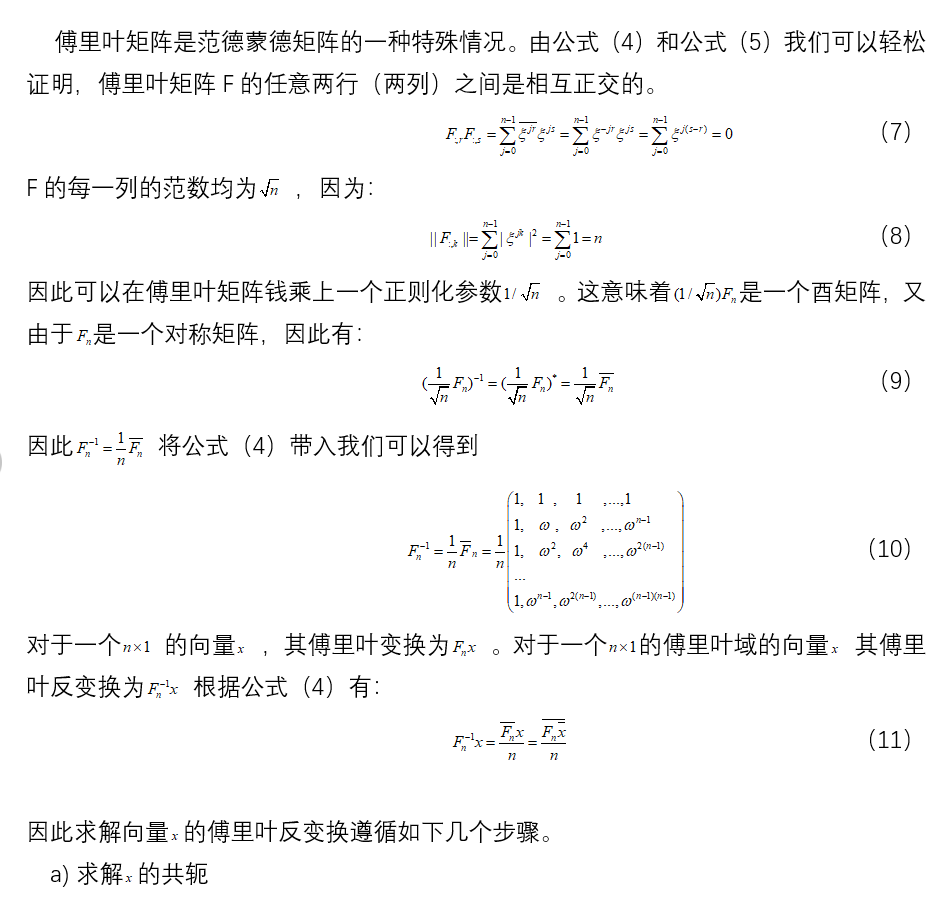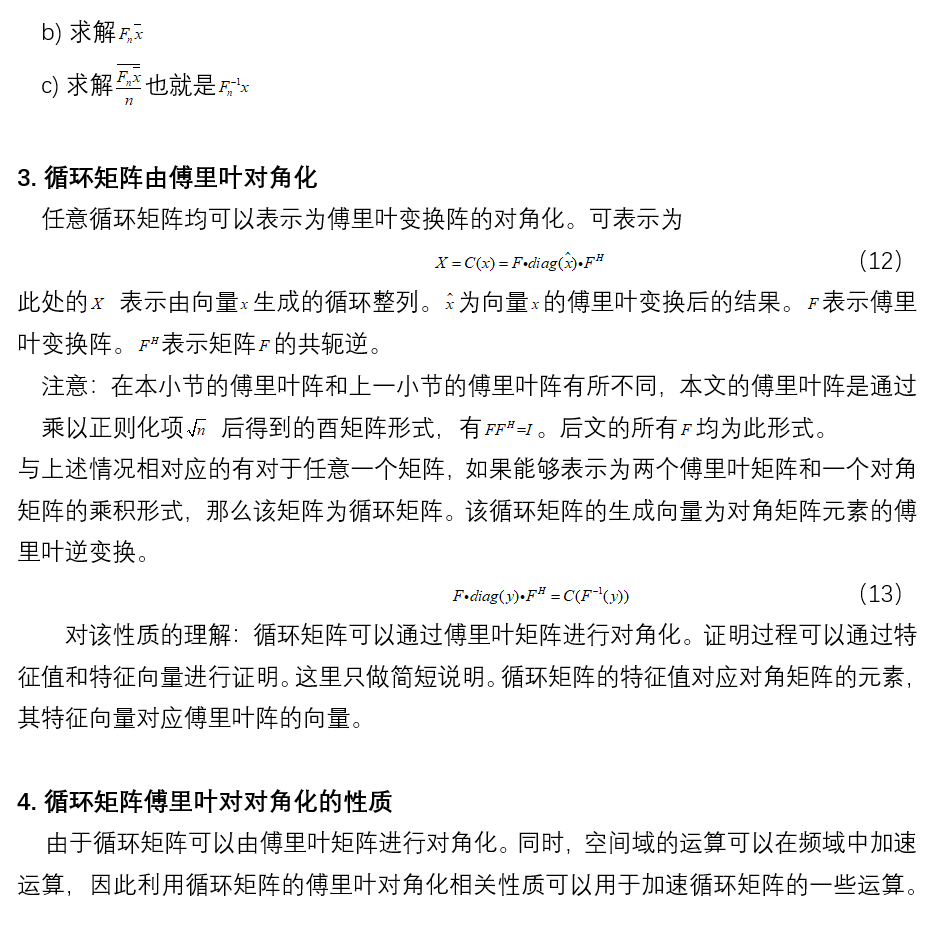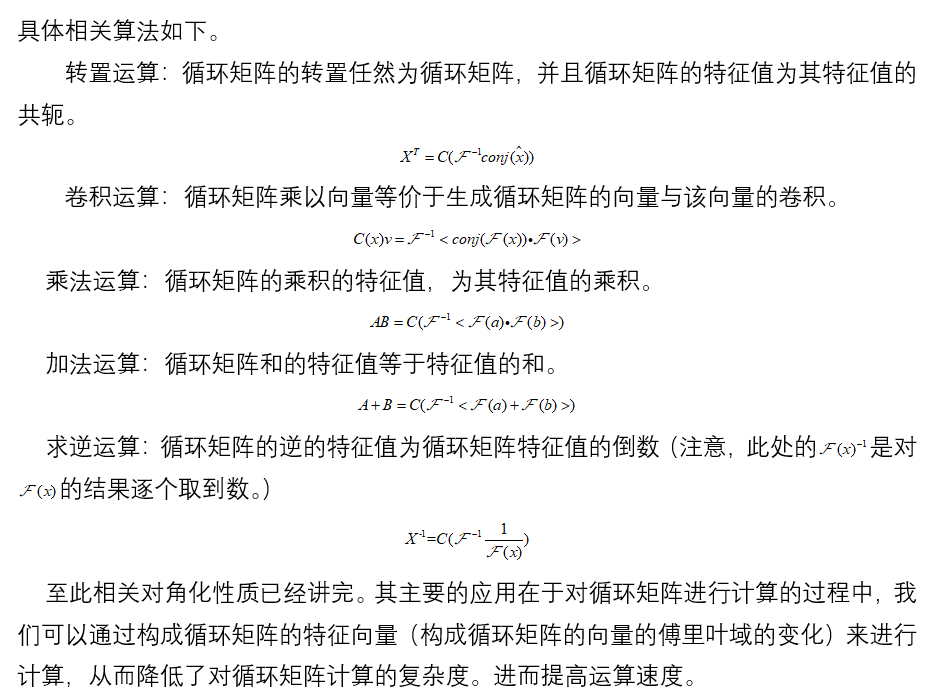文章类容根据《Matrix Analysis and Applied Linear Alebra》第5.8章节内容翻译。

展开全文• ## Toeplitz 矩阵对角化

千次阅读 2015-08-13 10:17:07
Toeplitz 矩阵是一种比较特殊的矩阵：其中任何一条对角线的元素取相同的值，
Toeplitz 矩阵是一种比较特殊的矩阵：其中任何一条对角线的元素取相同的值，即 An=⎡⎣⎢⎢⎢⎢⎢⎢⎢⎢⎢a0a1a2⋮ana−1a0a1⋮an−1a−2a−1a0⋱⋯⋯⋯⋱⋱a1a−na−n+1⋮a−1a0⎤⎦⎥⎥⎥⎥⎥⎥⎥⎥⎥<!--//--><![CDATA[//><!--
A_n = \left[
\begin{array}{ccccc}
a_{0}&  a_{-1} & a_{-2} & \cdots & a_{-n}\\
a_{1}&  a_{0} & a_{-1} & \cdots& a_{-n+1}\\
a_{2}&  a_{1} & a_{0} & \ddots& \vdots\\
\vdots& \vdots&\ddots &\ddots&a_{-1}\\
a_{n}&  a_{n-1} &\cdots & a_1& a_0 \\
\end{array}
\right]
//--><!]]>
这里不再展开证明。
Toeplitz 矩阵可以被Fourier矩阵对角化，即有 An=FHnΛFn<!--//--><![CDATA[//><!--
A_n=F_n^H \Lambda  F_n
//--><!]]>F<!--//--><![CDATA[//><!--
F
//--><!]]>的元素为 [Fn]ij=1n√ej2πik/n,0<=i,j<=n−1<!--//--><![CDATA[//><!--
[F_n]_{ij}=\frac{1}{{\sqrt {n} }}{e^{j2\pi ik/n}}, \quad 0<=i,j<=n-1
//--><!]]>Λ<!--//--><![CDATA[//><!--
\Lambda
//--><!]]>是n阶对角矩阵。

生成一个循环矩阵

 T = toeplitz([2 1 0 0 1]);

An=⎡⎣⎢⎢⎢⎢⎢⎢2100112100012100012110012⎤⎦⎥⎥⎥⎥⎥⎥<!--//--><![CDATA[//><!--
A_n = \left[
\begin{array}{ccc}
2 &    1&     0&     0&     1\\
1 &    2&     1&     0&     0\\
0 &    1&     2&     1&     0\\
0 &    0&     1&     2&     1\\
1 &    0&     0&     1&     2
\end{array}
\right]
//--><!]]>生成Fourier矩阵

F = dftmtx(5)

F=⎡⎣⎢⎢⎢⎢⎢⎢1.00001.00001.00001.00001.00001.00000.3090−0.9511i−0.8090−0.5878i−0.8090+0.5878i0.3090+0.9511i1.0000−0.8090−0.5878i0.3090+0.9511i0.3090−0.9511i−0.8090+0.5878i1.0000−0.8090+0.5878i0.3090−0.9511i0.3090+0.9511i−0.8090−0.5878i1.00000.3090+0.9511i−0.8090+0.5878i−0.8090−0.5878i0.3090−0.9511i⎤⎦⎥⎥⎥⎥⎥⎥<!--//--><![CDATA[//><!--
F = \left[
\begin{array}{ccccc}
1.0000&             1.0000&             1.0000&             1.0000&             1.0000\\
1.0000&             0.3090 - 0.9511i&  -0.8090 - 0.5878i&  -0.8090 + 0.5878i&   0.3090 + 0.9511i\\
1.0000&            -0.8090 - 0.5878i&   0.3090 + 0.9511i&   0.3090 - 0.9511i&  -0.8090 + 0.5878i\\
1.0000&            -0.8090 + 0.5878i&   0.3090 - 0.9511i&   0.3090 + 0.9511i&  -0.8090 - 0.5878i\\
1.0000&             0.3090 + 0.9511i&  -0.8090 + 0.5878i&  -0.8090 - 0.5878i&   0.3090 - 0.9511i
\end{array}
\right]
//--><!]]>对角化

Λ=F∗T∗conj(F)/5;<!--//--><![CDATA[//><!--
\Lambda = F*T*conj(F)/5;
//--><!]]>

Λ=⎡⎣⎢⎢⎢⎢⎢⎢4.000000000−0.0000i2.6180+0.0000i−0.0000+0.0000i−0.0000−0.0000i0.0000−0.0000i00.00000.38200.0000−0.00000−0.00000.00000.38200.00000+0.0000i0.0000+0.0000i−0.0000+0.0000i−0.0000−0.0000i2.6180−0.0000i⎤⎦⎥⎥⎥⎥⎥⎥<!--//--><![CDATA[//><!--
\Lambda = \left[
\begin{array}{ccccc}
4.0000 &                 0 - 0.0000i&        0&                  0&                  0 + 0.0000i\\
0  &           2.6180 + 0.0000i&   0.0000&            -0.0000&             0.0000 + 0.0000i\\
0  &          -0.0000 + 0.0000i&   0.3820&             0.0000&            -0.0000 + 0.0000i\\
0  &          -0.0000 - 0.0000i&   0.0000&             0.3820&            -0.0000 - 0.0000i\\
0  &           0.0000 - 0.0000i&  -0.0000&             0.0000&             2.6180 - 0.0000i
\end{array}
\right]
//--><!]]>
对角线上的元素便是Toeplitz 矩阵的特征值。
其实也可以用矩阵An<!--//--><![CDATA[//><!--
A_n
//--><!]]>的第一列变换得到

fft([2 1 0 0 1])

ans=[4.00002.61800.38200.38202.6180]<!--//--><![CDATA[//><!--
ans =
\begin{array}{ccccc}
[4.0000&    2.6180&   0.3820&    0.3820&    2.6180]
\end{array}
//--><!]]>
又或者这样得到

F*[2 1 0 0 1]'

ans=⎡⎣⎢⎢⎢⎢⎢⎢4.00002.61800.38200.38202.6180⎤⎦⎥⎥⎥⎥⎥⎥<!--//--><![CDATA[//><!--
ans =\left[
\begin{array}{ccccc}
4.0000\\
2.6180\\
0.3820\\
0.3820\\
2.6180
\end{array}
\right]
//--><!]]>另一个角度去理解频率域

空间域，两个序列的x,y<!--//--><![CDATA[//><!--
x,y
//--><!]]>卷积，等于其中一个序列排成的Toeplitz<!--//--><![CDATA[//><!--
\text{Toeplitz}
//--><!]]>矩阵乘以另一个序列，即Toep(x)⋅y<!--//--><![CDATA[//><!--
\text{Toep}(x) \cdot y
//--><!]]>。
空间域卷积的结果，等于两个序列各自的傅里叶变换X，Y<!--//--><![CDATA[//><!--
X，Y
//--><!]]>之积，即 Toep(x)⋅y=F−1⋅Diag(F⋅x)⋅F⋅y<!--//--><![CDATA[//><!--
\text{Toep}(x) \cdot y = F^{-1} \cdot \text{Diag}(F\cdot x) \cdot F \cdot y
//--><!]]>Toep(x)=F−1⋅Diag(X)⋅F<!--//--><![CDATA[//><!--
\text{Toep}(x)  = F^{-1} \cdot \text{Diag}(X) \cdot F
//--><!]]>F⋅Toep(x)⋅F−1=Diag(X)<!--//--><![CDATA[//><!--
F \cdot \text{Toep}(x) \cdot F^{-1} =\text{Diag}(X)
//--><!]]>
这里用Diag(X)<!--//--><![CDATA[//><!--
\text{Diag}(X)
//--><!]]>表示以向量X<!--//--><![CDATA[//><!--
X
//--><!]]>的元素为对角元的对角矩阵，Toep(x)<!--//--><![CDATA[//><!--
\text{Toep}(x)
//--><!]]>表示用向量x的元素排成的Toeplitz矩阵。即Fourier矩阵将Toeplitz矩阵对角化了。

参考

知乎，图像处理中，空间域的卷积相当于频率域的乘积，从矩阵分析角度如何理解？
展开全文图像处理
• 实对称矩阵的PPP除了满足可逆之外，还是正交矩阵 特征值篇4——实对称矩阵的特殊性 正规矩阵的PPP除了满足可逆之外，还是酉矩阵 酉相似于对角阵的充要条件
下面给出矩阵可相似对角化的充要条件：摘自 Linear Algebra and its applications David C. Lay
Chapter 5.3 , page282
矩阵相似对角化示例1矩阵相似对角化示例2实对称矩阵的$P$除了满足可逆之外，还是正交矩阵
特征值篇4——实对称矩阵的特殊性
正规矩阵的$P$除了满足可逆之外，还是酉矩阵
酉相似于对角阵的充要条件


展开全文• 1可以从不同角度（简单角度）去描述复杂问题 2.计算
• ## 循环矩阵

千次阅读 2016-12-05 22:46:06
今天讲讲线性代数中的一类特殊的矩阵———循环矩阵。形如：∥∥∥∥∥∥∥a1anan−1⋯a2a2a1an⋯a3a3a2a31⋯a4⋯⋯⋯⋯⋯anan−1an−2⋯a1∥∥∥∥∥∥∥\begin{Vmatrix} a_1&a_2&a_3 &\cdots &a_n \\ a_n&a_1&a_...
• 假设两个实对称矩阵A和B,如果存在一个可逆的矩阵X， XAX'=B,已知A和B,知道怎么用matlab求X? 本例中数据如下： A=[0.287402 0 0  0 0.483209 0  0 0 0.000025]; B=[0.287402 -0.028039 -0.0000727  -0....
• ## matlab循环矩阵

千次阅读 2017-03-17 18:45:19
• 矩阵按照对角线的数据累加，并且把结果的一边转换为一个三角形矩阵，在主程序调用怎么实现？
• 循环矩阵对角化及其性质在后面有简单介绍。 为化简计算，将输入X表示为循环矩阵的形式，则 X H X X^HX 的特征值为 x ^ ⨀ x ^ ∗ \hat{x}\bigodot\hat{x}^* , I 本身就是一个循环矩阵，其生成向量记为 δ \...机器学习
• public class DoubleArray { public static void main(String args[]) { int a[][] = new int; //创建矩阵 for(int i = 0 ; i <= 5 ;i++) { //给矩阵元素赋值 fo...java
• 引用：http://www.ilovematlab.cn/thread-74502-1-1.html 如：A=[ 1 2 3  2 3 4]  B=[1 2  3 4 ] 得到一个C=[A 0  0 B]; 在matlab中可以使用blkdiag命令 C = blkdmatlab 合并
• 在Matlab中不用eig()命令求方阵的特征值和特征向量矩阵，并判断其是否可以对角化 #本文仅针对标题所示问题的代码解答，若相关概念不熟，请去资料。 #不要问我为什么放着好好的eig()命令不用，反而去写一堆代码。这得...matlab 线性代数
• 循环矩阵充分利用循环矩阵及其特性的是核相关滤波跟踪算法的另一个重要特征，它不仅涉及到目标采样，而且巧妙的将目标特征的频域空间与岭回归相结合，实现了目标特征的快速学习与检测。首先考虑一维样本的情况，设x=...算法 相关滤波
• 循环矩阵 根据https://max.book118.com/html/2016/0519/43353557.shtm整理修订 1.循环矩阵的定义 定义1 数域P\mathbb{P}P上的n×nn \times nn×n矩阵 Cn=circ(c0,c1,⋯ ,cn−1)=(c0c1c2⋯cn−1cn−1cn−1c0c1⋯cn...
• 文章目录一、三对角方程数值解(Thomas algorithm或称“追赶法”)二、循环对角方程数值解(Sherman-Morrison formula)三、参考文献 一、三对角方程数值解(Thomas algorithm或称“追赶法”) %{ Function: solve_...Thomas算法 追赶法matlab
• // 利用数组输出如下图所示的3*3矩阵，并求该矩阵对角线元素之和 double arr[][] ={{3,5,2.5},{4,7,6},{8,9,10}}; //遍历二维数组 for(int i=0;i<arr.length;i++){//遍历每个元素里面的数组 for(int...javabase
• 最常见的循环莫过于对矩阵中的每一个元素进行操作，对于编程思维还在C语言或者C++，JAVA的人来说，第一反应就是两层循环，先来个 “for i=1：m”对矩阵的行进行循环，再来个“for j=1：n”对矩阵的列进行循环。...
• 矩阵对角化引入探讨线性变换的对角化问题。（定义、等价命题和定理）线性代数 线性变换
• 注意，julia中没有diag函数，如果想要定义4*4的对角矩阵，可以使用下面方法： 1. 使用Matrix函数 using LinearAlgebra Matrix{Float64}(I,4,4) 注意，Matrix{Float64}(I,4,4)中是字母I，而不是数字1 ...
• ## matlab对角化

千次阅读 2017-03-17 18:23:30
A=[2 2 -2;2 5 -4;-2 -4 5] [V, D]= eig(A) D=inv(V)*A*V
• 编写一个算法，在非负矩阵中，从左上走到右下，每次只能向左或向下移动一格，输出走过的路径节点坐标和最小权值。 方法：动态规划法 状态转移方程stat[i][j] = min{stat[i-1][j], stat[i][j-1]}。 逻辑：目的...最短路径...